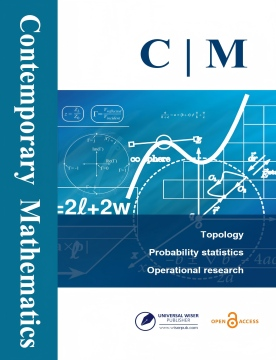# Contemporary Mathematics

ISSN: 2705-1064 (Print) 2705-1056 (Online) Description

Contemporary Mathematics is a quarterly peer-reviewed scientific journal. Our ultimate goal is to make the journal an important source for publishing high quality papers related to the development of contemporary mathematics as well as a continuing and evolving source of interesting and relevant problems for researchers.

The scope of this journal includes theoretical, numerical, and experimental studies in all fields of mathematics and the mathematical sciences. A broad spectrum of topics are covered: mathematical theory, pure mathematics, algebra, geometry and topology, complex analysis, differential equations, industrial mathematics, computational mathematics, discrete mathematics, applied mathematics, etc.

# Latest Articles

## Open AccessArticle

Extending the Convergence of Two Similar Sixth Order Schemes for Solving Equations under Generalized Conditions

by Ioannis K. Argyros, Santhosh George, Christopher I. Argyros

ABSTRACT: The applicability of two competing efficient sixth convergence order schemes is extended for solving Banach space valued equations. In previous works, the seventh derivative has been used not...

## Open AccessArticle

Extending the Applicability and Convergence Domain of a Fifth-Order Iterative Scheme under Hölder Continuous Derivative in Banach Spaces

by Debasis Sharma, Sanjaya Kumar Parhi, Shanta Kumari Sunanda

ABSTRACT: The most significant contribution made by this study is that the applicability and convergence domain of a fifth-order convergent nonlinear equation solver is extended. We use Hölder condition on...

## Open AccessArticle

On Global Inversion Theorems in the Plane

by Hong Ding

ABSTRACT:

## Open AccessArticle

Construction of Brauer-Severi Varieties

by Elisa Lorenzo García

ABSTRACT: In this paper, we give an algorithm for computing equations of Brauer-Severi varieties over fields of characteristic 0. As an example, we show the equations of all Brauer-Severi surfaces defined...

## Open AccessArticle

Global Solutions for the Kuramoto-Sivashinsky Equation Posed on Unbounded 3D Grooves

by N.A. Larkin

ABSTRACT: Initial boundary value problems for the three-dimensional Kuramoto-Sivashinsky equation posed on unbounded 3D grooves (that may serve as mathematical models for wildfires) were considered. The...

## Open AccessArticle

An Existence Result for a Class of Coupled Polyharmonic Systems

by Zeineb Ben Yahia, Zagharide Zine El Abidine

ABSTRACT: This work deals with the existence of positive continuous solutions for a nonlinear coupled polyharmonic system. Our analysis is based on some potential theory tools, properties of functions in...